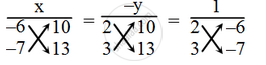# Solve the follownig system of equations by the method of cross-multiplication. 2x – 6y + 10 = 0; 3x – 7y + 13 = 0 - Mathematics

Sum

Solve the follownig system of equations by the method of cross-multiplication.

2x – 6y + 10 = 0

3x – 7y + 13 = 0

#### Solution

The given system of equations is

2x – 6y + 10 = 0 ….(1)

3x – 7y + 13 = 0 ….(2)

By cross-multiplication, we have\Rightarrow \frac{x}{-6\times 13-(-7)\times 10}=\frac{-y}{2\times13-3\times 10}=\frac{1}{2\times (-7)-3\times (-6)}

\Rightarrow \frac{x}{78+70}=\frac{-y}{26-30}=\frac{1}{-14+18}

\Rightarrow \frac{x}{-8}=\frac{-y}{-4}=\frac{1}{4}

\Rightarrow \frac { x }{ -8 } = \frac { 1 }{ 4 }

⇒ x = – 2

\Rightarrow \frac { -y }{ -4 } = \frac { 1 }{ 4 }

⇒ y = 1

Hence, the solution is x = – 2, y = 1

Concept: Algebraic Methods of Solving a Pair of Linear Equations - Cross - Multiplication Method
Is there an error in this question or solution?

Share